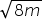Mathematics
Easy

Question

# A car skidded m ft during a race. The expression s =is the rate of speed of the car in ft/s. Find the rate of the speed of the car, if the car skidded 288 ft

## 12362448Hint:

## The correct answer is: 48

### Here, we have to find the speed of a car when it skidded 288m during a race given that s = √8m.We have, s = √8m=> s = √(8×288)=> s = √2304=> s = 48 ft/s.Hence, the correct option is D.

A radical expression is an expression containing a square root.

### Related Questions to study#### With Turito Foundation.#### Get an Expert Advice From Turito.